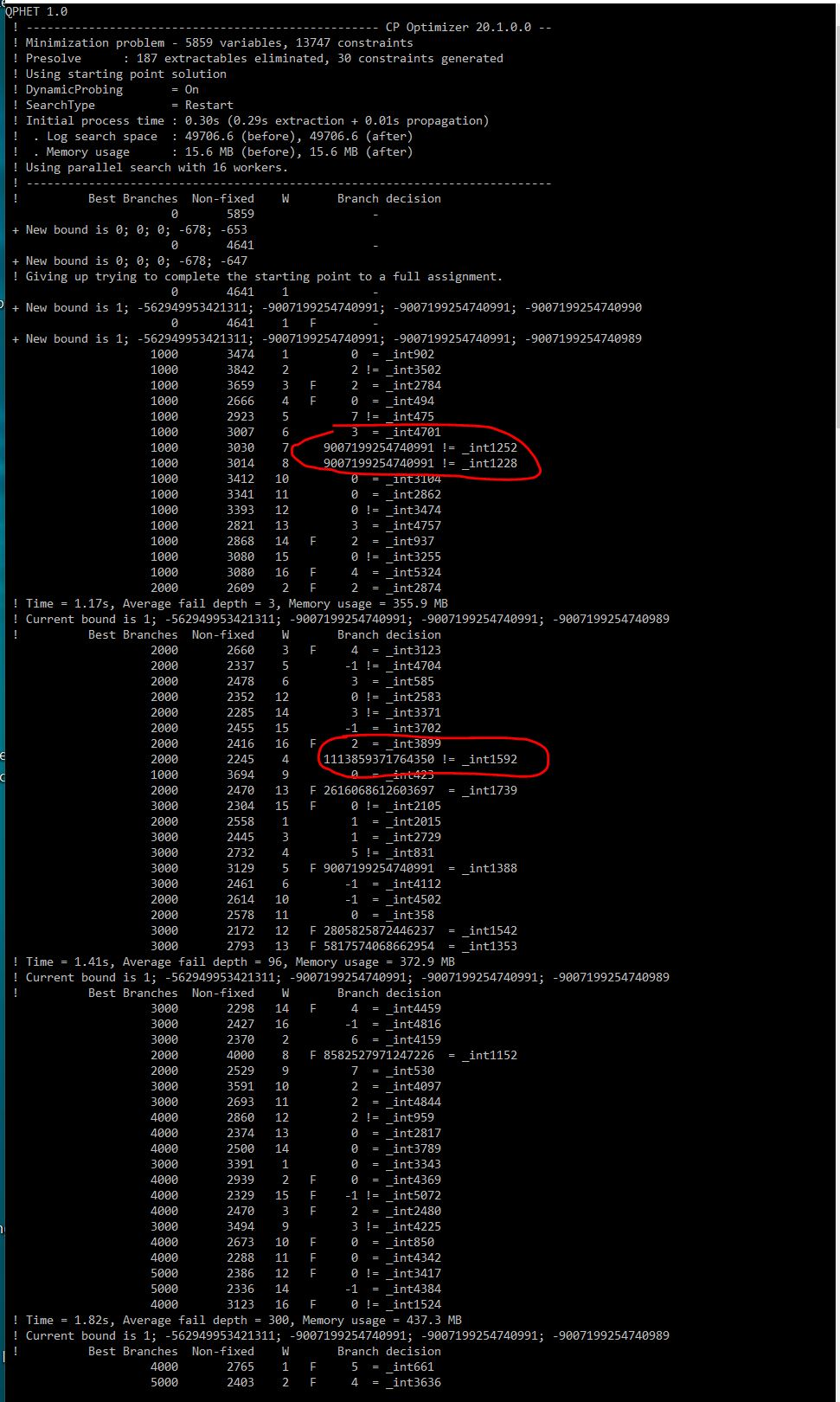# Decision Optimization

View Only

## Understanding log file#### javier rodrigoMon January 03, 2022 08:12 AM#### ALEX FLEISCHERMon January 03, 2022 10:49 AM• #### 1.  Understanding log file

Posted Mon January 03, 2022 08:12 AM
I am trying to debug my model because it is taking a long time without getting a solution and when I look at the log file I can see very large numbers that I don´t know where they come from. Are these large numbers caused by an error in the model (the small numbers are coherent with some of my variable possible values)? If so, is there any way that helps me troubleshooting the error (knowing which constraint is coming from)?

The model is done with C++ API. If needed I could provide the code although it's a bit long. Thanks------------------------------
javier rodrigo
------------------------------

• #### 2.  RE: Understanding log file

Posted Mon January 03, 2022 10:49 AM
Hi,

do you have unbounded decision variable such as

``dvar int x;​``

that you could turn into

``dvar int x in 0..20;``

in order to make the search space smaller ?

------------------------------
[Alex] [Fleischer]
[EMEA CPLEX Optimization Technical Sales]
[IBM]
------------------------------

• #### 3.  RE: Understanding log file

Posted Mon January 03, 2022 01:25 PM

``````        IloArray<IloIntVarArray> TurneroCalculado(env, simu.plantilla.size());
for (i = 0; i < simu.plantilla.size(); i++) {
TurneroCalculado[i] = IloIntVarArray(env, simu.numDiasTurnero, 0, numDeTurnos-1);
for (d = 0; d < simu.numDiasTurnero; d++) {
}
}​``````
and I thought I was setting the min and max of the TurneroCalculado[i][d] variables from 0 to numDeTurnos-1. When I changed to ...

``````        IloArray<IloIntVarArray> TurneroCalculado(env, simu.plantilla.size());
for (i = 0; i < simu.plantilla.size(); i++) {
TurneroCalculado[i] = IloIntVarArray(env, simu.numDiasTurnero, 0, numDeTurnos-1);
for (d = 0; d < simu.numDiasTurnero; d++) {
TurneroCalculado[i][d] = IloIntVar(env, 0, numDeTurnos - 1);
}
}​``````
is not giving those numbers

Still too slow but at least not giving those numbers. Thanks

------------------------------
javier rodrigo
------------------------------